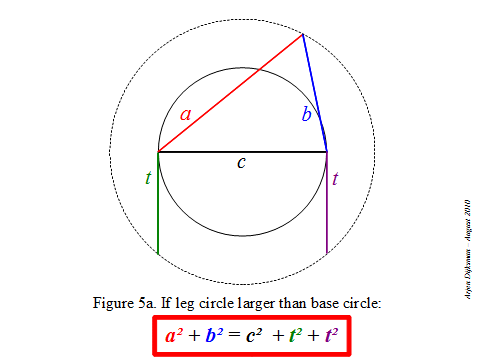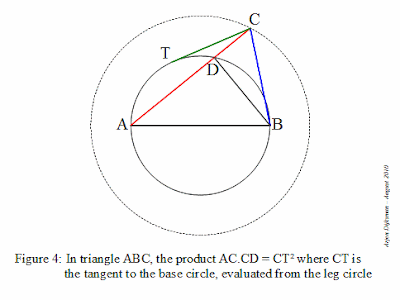## Saturday, August 28, 2010

### Alternative Pythagorean quadruples and other extensions to Pythagoras theorem

The vertices of an arbitrary triangle can be disposed onto two concentric circles such that the base is the diameter of the first circle (which I call the base circle) and the opposite vertex is on the second circle (which I call the leg circle). As there are three bases, there are generally three ways to arrange this setting. I mentioned in my preceding post that we can apply the Pythagorean-like relation a²+b²=c²±2t² to this triangle, where c is its base, a and b are the legs and t is the tangent to the inner circle emanating from the outer circle. For clarity, I reproduce an illustrative figure from my preceding post, for the case where the inner circle is the base circle.Now, like for the classical Pythagoras relation, there are many features that can be investigated regarding this alternative relation. For example, I would like to better understand the relation of this 2D formula with the Cartesian 3D variant of Pythagoras: x²+y²+z²=d², which is analogous to the case where the leg circle is smaller than the base circle (see Figure 5c of preceding post). Another interesting aspect is the fact that the square of the tangent (multiplied by π) determines the area of the annular ring delimited by the base and the leg circles, as stated by Mamikon Mnatsakanian. This fact offers possibilities for areal representations of the squares or circles related to the sides of the arbitrary triangle, like we are acquainted to do with the squares related to the sides of a right triangle. Also, what would be interesting to develop is its relation with the law of cosines (as noticed by Pat Ballew) and the angle subtended by sides a and b. I guess this will leave enough stuff for future posts or conversations, or even papers in specialized journals.

As a conclusion, let me mention Pythagorean numerological features which provide stuff for entertaining puzzles. Maybe you know the Pythagorean triples, those sets of integer numbers (n,m,l) that verify n²+m²=l² and of which (3,4,5) is the simplest instance. The 3D Pythagoras relation allows an extension to Pythagorean quadruples, sets of integer numbers (n,m,l,k) that satisfy n²+m²+l²=k² and of which (1,2,2,3) is the simplest instance. I couldn't resist to look for some integer quadruples that satisfy a²+b²=c²+2t². Among them I found two nice quadruples with successive a,b,c :

(7,8,9,4) for which 7² + 8² = 9² + 2 × 4² = 113

(35,36,37,24) for which 35² + 36² = 37² + 2 × 24² = 2521

## Sunday, August 15, 2010

### A Pythagorean relation for any triangle?

Physics makes extensive use of the Pythagorean law relating the squares of the sides of a right triangle. The well-known a² + b² = c² relation is of special interest for the determination of distances and lengths of vectors, but also for energy conservation laws and Lorentz transformations. There are various relations that resemble the Pythagoras law, but none of them seems to have the usefulness of Pythagoras’ original one, as well as the “beauty” originating from its sole quadratic terms. When the vertex opposite to the hypotenuse runs on the circle determined by the right triangle, we have the Pythagoras relation illustrated in Figure 1.
Some time ago, I was made aware of another interesting Pythagorean law, discovered by Nguyen Tan Tai and which I mentioned in a previous post. For any triangle with sides a, b and c, if the vertex opposite to the base (say c) runs on any fixed circle centered at the center of c, we have the relation:

a² + b² = c² + constant

In order to have a better understanding of this quadratic relation, I tried to re-derive it in my own mental representation. I will call the fixed circle with the running vertex C the “leg circle”, because it is determined by the vertex that is common to legs AC and BC of the triangle (see Figure 2).When the leg circle is larger than the base circle, the legs AC and BC intersect the base circle and we can decompose the arbitrary triangle into two right triangles, for example as illustrated in Figure 3, the right triangles ABD and BCD.With the Pythagoras relation we than have:

AC² + BC² = (AD + CD)² + BC²
= (AD² + CD² + 2.AD.CD) + (BD² + CD²)
= AD² + BD² + 2.CD² + 2.AD.CD
= (AD² + BD²) + 2.(AD + CD).CD
= AB² + 2.AC.CD

But the product AC.CD is the power of point C with respect to the base circle, i.e. for every point C on the leg circle, AC.CD is constant and equal to the square of the tangent ray CT (see Figure 4).If we use another notation, AC = a, BC = b, AB = c and CT = t, the relation becomes a nice equation with only quadratic terms:

a² + b² = c² + t² + t²

One can verify that if the leg circle has same size as the base circle, we retrieve the original Pythagoras relation :

a² + b² = c²

And if the leg circle is smaller than the base circle, we have:

a² + b² + t² + t² = c²

where t is now the tangent ray to the leg circle emanating from any point of the base circle.

We thus have a Pythagoras-like relation for any triangle given its base and leg circles, as illustrated by Figures 5a, 5b and 5c.